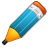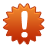# Programming/Python

## Programming Details

Python is a widely used high-level programming language used for general-purpose programming.

An interpreted language, Python has a design philosophy which emphasises code readability (notably using white-space indentation to delimit code blocks rather than curly braces or keywords), and a syntax which allows programmers to express concepts in fewer lines of code than possible in languages such as C++ or JAVAWhen programming with Python in a HPC environment you will need to change the first line as shown below
```
#!/usr/bin/python

```

To

```
#!/usr/bin/env python

```

#### Python example

```
#!/usr/bin/env python

from mpi4py import MPI

comm = MPI.COMM_WORLD
rank = comm.Get_rank()

if rank == 0:
data = {'key1' : [7, 2.72, 2+3j],
'key2' : ( 'abc', 'xyz')}
else:
data = None

data = comm.bcast(data, root=0)

if rank != 0:
print ("data is %s and %d" % (data,rank))
else:
print ("I am master\n")
```Due to the limited of the Python interpreter (GIL) there is no point to use threads for CPU intensive tasks in Python. You need either multiprocessing or use C extensions that release GIL during computations e.g., some of numpy functions, example.

#### Modules Available

The following modules are available:

Python provided by the Python Software foundation

Anaconda python Anaconda is the open data science platform powered by Continuum

#### Compilation

Python is byte compiled at runtime by typing for example

```

```

## Usage Examples

### Batch example

```
#!/bin/bash
#SBATCH -J compute-single-node
#SBATCH -N 1
#SBATCH -o %N.%j.%a.out
#SBATCH -e %N.%j.%a.err
#SBATCH -p compute
#SBATCH --exclusive

echo \$SLURM_JOB_NODELIST

module purge

export I_MPI_DEBUG=5
export I_MPI_FABRICS=shm:tmi
export I_MPI_FALLBACK=no

```

```[username@login01 ~]\$ sbatch python-demo.job
Submitted batch job 289572
```

## Python and OpenMP

The Python interpreter uses a GIL (Global Interpreter Lock) which makes multi-threading almost impossible. Although there are ways around this and one of the most common methods is to use Cython.

Cython supports native parallelism through the cython.parallel module. To use this kind of parallelism, the GIL must be released (see Releasing the GIL). It currently supports OpenMP

• Example with a reduction (on sum):
```
#!/usr/bin/env python
from cython.parallel import prange

cdef int i
cdef int n = 30
cdef int sum = 0

for i in prange(n, nogil=True):
sum += i

print(sum)
```
• Example with a typed memoryview (e.g. a NumPy array):
```
#!/usr/bin/env python
from cython.parallel import prange

def func(double[:] x, double alpha):
cdef Py_ssize_t i

for i in prange(x.shape):
x[i] = alpha * x[i]
```

To actually use the OpenMP support, you need to tell the C or C++ compiler to enable OpenMP. For gcc this can be done as follows in a setup.py:

```
#!/usr/bin/env python
from distutils.core import setup
from distutils.extension import Extension
from Cython.Build import cythonize

ext_modules = [
Extension(
"hello",
["hello.pyx"],
extra_compile_args=['-fopenmp'],